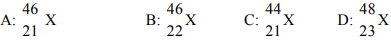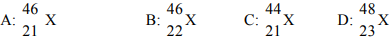# Problem: Which of the following pairs represent (hypothetical) isotopes? A) A and BB) A and CC) A and DD) C and D

###### FREE Expert Solution

We are asked to identify the pairs which are hypothetical isotopes from below:Recall that Isotopes represent atoms of an element that contain the same atomic number (same number of protons), but different mass numbers (different number of neutrons).

79% (207 ratings)###### Problem Details

Which of the following pairs represent (hypothetical) isotopes?A) A and B
B) A and C
C) A and D
D) C and D